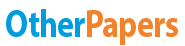Gross Fixed Capital Formation Per Capita

Essay by   •  May 19, 2019  •  Research Paper  •  4,151 Words (17 Pages)  •  57 Views

Essay Preview: Gross Fixed Capital Formation Per Capita

Report this essay
Page 1 of 17

PROJECT

UNIVERSITY OF ECONOMIC STUDIES

International Business And Economics

Iuga Cristiana Elena

Group 939

[pic 1]

1. Introduction

The object of this project is to show the relationship between 2 variables. In my case, the first variable is represented by the: “ GDP per capita” measured in \$ and the second one is: “Gross Fixed Capital Formation per capita” measured also in \$.

Definitions

The first variable is represented by GDP per capita. The GDP is the gross domestic product of a country. Gross domestic product is the amount of goods and services produced inside a country. This is an economic measurement used to compare two countries. The gross domestic product per capital is the average amount an individual contributed toward the gross domestic product. The formula for gross domestic product per capital is the gross domestic product of a country divided by the population of the country. Usually is measured in \$.

The second variable is Gross fixed capital formation per capita (formerly gross domestic fixed investment) includes land improvements (fences, ditches, drains, and so on); plant, machinery, and equipment purchases; and the construction of roads, railways, and the like, including schools, offices, hospitals, private residential dwellings, and commercial and industrial buildings. According to the 1993 SNA, net acquisitions of valuables are also considered capital formation. Data are in current U.S. dollars. Per \$ GDP figures expressed per 1 \$ gross domestic product.

The countries analized in this project are included in 3 regions:

a)        European Union

b)        Western Balkans

c)        North America

I had collected the data from: UNECE Statistical Database, compiled from national and international (CIS, EUROSTAT, IMF, OECD, World Bank) official sources. With these data I want to do more statistical problems.

1. European Union-Table1

[pic 2]

B) Western Balkans-Table2

[pic 3]

[pic 4]

[pic 5]

C) North America-Table3

[pic 6]

[pic 7]

Source of all 3 tables: UNECE Statistical Database, compiled from national and international (CIS, EUROSTAT, IMF, OECD, World Bank)

Assumptions:

Problems encountered+ solutions

I found a country that repeats twice so I decided to eliminate it because it affected my calculation result, considering it twice. The one in question is called Croatia and is part of both the UE and the Western Balkans.

1. Raw Data( Mean, Median, Mode)

Mean

For ungrouped data, the mean is the sum of all the observations divided by the total number of observations.

[pic 8]

For Variable 1:

30,001.11 \$[pic 9]

For Variable 2:

6,139.80 \$[pic 10]

Interpretation:

The average GDP per capita of the 35th countries is 30,001.11\$.

The average Gross Fixed Capital Formation per capita of the 35th countries is 6,139.80 \$.

Median

For ungrouped data, the median can be calculated as follows:

If the data contains an odd number of the observations, the median is the middle number, which is (n+1)/2 ordered observation.

N=35

Median location is: (35+1)/2=36/2=18

After I sorted the data, I obtained:

For Variable 1:

The 18th position is represented by: Malta-28,580\$

For Variable 2:

The 18th position is represented by: Slovenia- 5,883\$

Interpretation:

Half of the GDP per capita (Variable 1) is under 28,580\$ and half above 28,580\$.

Half of the Gross Fixed Capital Formation per capita (Variable2) is under 5,883\$ and half above 5,883\$.

Mode

For ungrouped data, to determine the mode, we only need to observe the value with the highest frequency.

Because for both variables I didn’t found a value that repeated twice, I assume that there didn’t exist the Mode.

1. Raw Data - Measures of Variability

The Range

The absolute range of a set of observations is the difference between the largest and the smallest observations:

R= xmax-xmin

For Variable 1: R=85,392-8,942=76,450\$

For Variable 2: R=15,019-1,517=13,502\$

Interpretation: The difference between the largest and the smallest in case of:

1. Variable 1 is 76,450\$
2. Variable 2 is 13,502\$

The Relative Range

Is calculated as follows:

R(%)=*100[pic 11]

For Variable 1:

R(%)=(76,450/30,001.11)*100=254.82 %

For Variable 2:

R(%)=(13,502/ 6,139.8)*100= 219.91%

Interpretations:

Because for both variables, the relative average is bigger than 100-120% (where the data series is homogenous), so I assume that the data series are not homogenous, they are not representative.

The Variance

It is a computed measure of variance whose value is affected by the value of every observation is a series and therefore this measure reflects the dispersion of all the observations.

σ2=[pic 12]

Because the numbers are very large, I used the function: var.p(variable_x)

Variable 1:

σ2=Var.p(variable1)= 216,748,429.07\$

Variable 2:

σ2=Var.p(variable2)= 7,509,000.45\$

Interpretations:

Because the results are huge in both cases, there exists extreme variability in data set.

The Standard Deviation

The standard of deviation of a set of observation is the most frequently encountered measure of dispersion and is calculated as the square root of the variance.

...

...

Download as:   txt (28 Kb)   pdf (2.2 Mb)   docx (1.3 Mb)
Continue for 16 more pages »
Only available on OtherPapers.com
Citation Generator

(2019, 05). Gross Fixed Capital Formation Per Capita. OtherPapers.com. Retrieved 05, 2019, from https://www.otherpapers.com/essay/Gross-Fixed-Capital-Formation-Per-Capita/65896.html

"Gross Fixed Capital Formation Per Capita" OtherPapers.com. 05 2019. 2019. 05 2019 <https://www.otherpapers.com/essay/Gross-Fixed-Capital-Formation-Per-Capita/65896.html>.

"Gross Fixed Capital Formation Per Capita." OtherPapers.com. OtherPapers.com, 05 2019. Web. 05 2019. <https://www.otherpapers.com/essay/Gross-Fixed-Capital-Formation-Per-Capita/65896.html>.

"Gross Fixed Capital Formation Per Capita." OtherPapers.com. 05, 2019. Accessed 05, 2019. https://www.otherpapers.com/essay/Gross-Fixed-Capital-Formation-Per-Capita/65896.html.